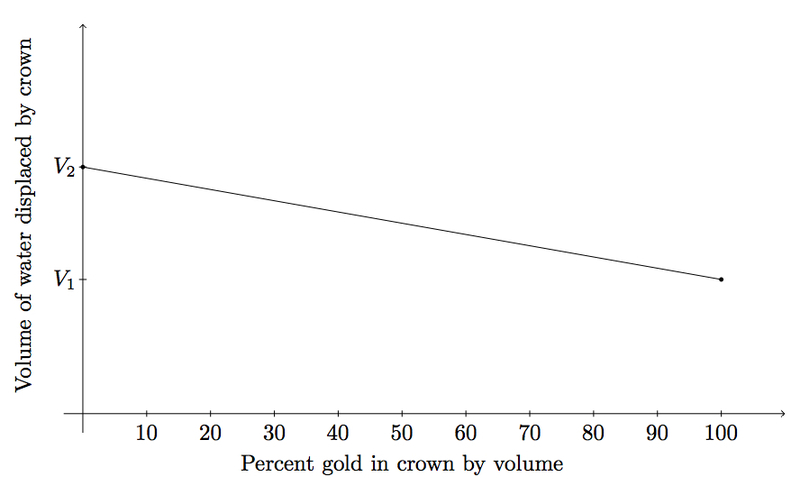# Archimedes and the King's crown

Alignments to Content Standards: G-MG.A.2

The King of Syracuse reportedly requested Archimedes' advice for determining if a crown was made with the appropriate mixture of gold and silver. Archimedes devised a method requiring the following measurements:

• the volume $V$ of water displaced by the crown when it is submerged in water,
• the volume $V_1$ of water displaced by an equal mass (that is, equal to the mass of the crown) of gold when submerged in the water,
• the volume $V_2$ of water displaced by an equal mass of silver when submerged in water.
1. How much water would a solid gold crown displace? What about a solid silver crown? What if the crown is half gold (by volume) and half silver (by volume)?
2. If $p$ denotes the percentage of the crown, by volume, which comes from gold, how much water will the crown displace?
3. Show that Archimedes' method can always determine the percentage of gold in the crown from its mass and volume.

## IM Commentary

The famous story of Archimedes running through the streets of Syracuse (in Sicily during the third century bc) shouting ''Eureka!!!'' (I have found it) reportedly occurred after he solved this problem. The problem combines the ideas of ratio and proportion within the context of density of matter. In practice, Archimedes' method requires knowing that the crown is made of gold and silver alone. If a merchant were to insert lead, a much cheaper but also very dense material, the argument would no longer work as there would be a third volume $V_3$ involved and a more complicated algebraic expression relating the composition of the crown to its density. The teacher may wish to share the picture at the beginning of the solution with students to help them visualize Archimedes' idea.

The problem asks students to find the amount of water displaced given the relative percentages of silver and gold by volume in the crown. The question could also be formulated in terms of the relative percentages of silver and gold by mass . The structure of the argument would not change but the graph would no longer be linear in part (c).

More generally, this method of relating volumes and density relates to other experiences in students' lives. For example, the tremendous force required to submerge an inner tube filled with air under water comes from the fact that the air occupies a lot of space with very little density. Submerging the tube means displacing a large volume of water and of course water is relatively dense. Teachers may also wish to discuss, in this same regard, how it is that an aircraft carrier, whose weight is phenomenally large, is able to float on water.

Though it is not possible to know with certainty how Archimedes made his discovery, one likely possibility is that he took a balance with the crown on one side and an equal mass of gold on the other and then submerged the entire balance in water. If the two sides remained balanced, then the crown was made of solid gold. If not, then the lighter material in the crown would displace more water and the submerged balance would tilt toward the side with the gold.

## Solution

A picture showing how to find the volume of the crown is given below:Measuring the two water levels indicated and subtracting gives the volume of the crown which is the same as the volume of the water displaced when it is submerged.

1. We are given that a solid gold crown would displace a volume $V_1$ of water while a solid silver crown would displace a volume $V_2$ of water. If the crown is half silver and half gold by volume, the silver half would displace $\frac{V_2}{2}$ and the gold half would displace $\frac{V_1}{2}$. So the total amount of water displaced by a crown which is half silver and half gold would be $$\frac{V_1 + V_2}{2}.$$
2. Reasoning as in part (a), this crown would contain a percent $p$ of a solid gold crown and $1-p$ of a solid silver crown. Therefore the amount of water displaced would be $$pV_1 + (1-p)V_2.$$ Note that this agrees with the answer in part (a) which deals with the cases where $p = 1,0,\frac{1}{2}$.

3. First note that gold is almost twice as dense as silver and so an equal mass of gold will displace less water. This means that $V_1$ is smaller than $V_2$. As the percent $p$ of gold, by volume, in the crown varies, from $0$ to $100$ the volume of water displaced will vary from $V_2$ to $V_1$ in the manner described in part (b). This is graphed below:Note that each percent $p$ corresponds to precisely one volume $V$ between $V_1$ and $V_2$. Therefore, once Archimedes has found the volume $V$ of water displaced by the crown, the graph will indicate percent of gold and silver in the crown: this is equivalent to solving the algebraic equation $$pV_1 + (1-p)V_2 = V$$ for $p$. We can rewrite this as $$p(V_1 - V_2) = V - V_2$$ and so the proportion of gold, by volume, in the crown is given by $$p = \frac{V-V_2}{V_1 - V_2}.$$